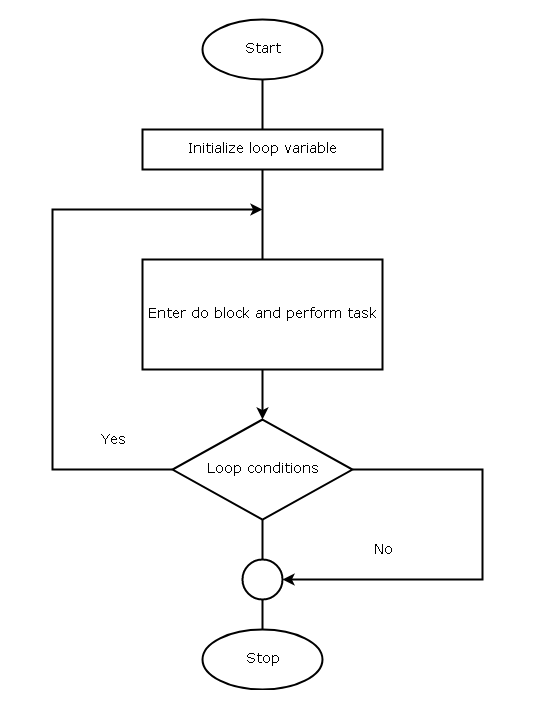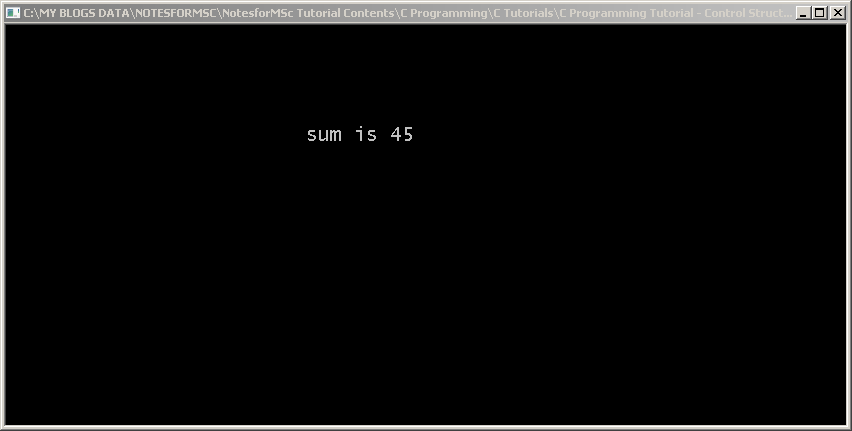## C Programming Tutorial – Do-While Loop

The  C do-while loop is a different kind of loop which checks for exit conditions instead of entry condition. So it is very useful if you want a loop that keeps iterating until an exit criterion is met.

In a C program, the do-while loop will let you execute your code first and then checks for test condition if it is true to complete the execution.

If the test condition is false, the loop terminates and ignores any computation it may have performed previously.

## Diagram for C Do-While Loop

The diagram below is used for a Do-While loop when we create a flowchart for the C program.Flowchart – C Do-While Loop

## Example Program

``````#include <stdio.h>

int main ()

{

int i, sum;

/* Initialization of loop variable 'i' and sum */

i = 0;

sum = 0;

/* Do-while loop body execution starts */

do {

sum = sum + i;

/* Increment the loop */

i++;

} while (i < 10); /* check for exit condition */

printf ("\n\n\n\n\n");

printf ("\t\t\tThe sum is %d", sum);

getch ();

return 0;

}
``````

## Output – C Do-While LoopOutput – C Do While

## Example Explained

In the above example, the do-while is initialized like other loop control structures – for and while loop. The variable sum is also initialized to 0.

``````i=0;
sum = 0;
``````

Then, the do block is started with executing the following statement. Unlike other loops, the do-while does not check for entry conditions and directly execute the loop body.

``````sum = sum + i;
``````

Next, the do-while loop is incremented for next iteration.

``````i++;
``````

When all the tasks in a do block is completed the while condition checks for the Boolean value of the loop and decides to continue if true or terminates the do-while loop if false.

The program then prints the output, when the loop terminates.

``````printf (“The sum is %d”, sum);
``````

and the program ends.

### Bibliography

Balagurusamy, E. 2000. Programming in ANSI C. Tata McGraw-Hill Education,.

Kanetkar, Yashavant. 20 November 2002. Let us C. Bpb Publications.